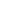DASCA is the World’s Foremost Standards & Credentialing Body for the Data Science Profession.

Compensation Trends

# Insights

## Compensation TrendsFoote Partners, the Florida-based IT consulting firm, reflects interesting figures on the premium you can expect to earn (or pay-out) if you’re in Big Data. It released the results in its 2016 IT Skills Demand and Pay Trends Report, which looks at the pay premiums being paid for the top skill areas in data management and data analytics. Here is what you can expect to earn, or pay out, in extra compensation for having the top data skills.

Data Architecture
Median = 19% Low (10 Percentile) = 16% High (90 Percentile) = 21%

Prescriptive Analytics
Median = 17% Low (10 Percentile) = 14% High (90 Percentile) = 19%

Big Data Analytics
Median = 17% Low (10 Percentile) = 14% High (90 Percentile) = 18%

Data Analytics
Median = 16% Low (10 Percentile) = 14% High (90 Percentile) = 18%

Predictive Analytics and Modeling
Median = 15% Low (10 Percentile) = 13% High (90 Percentile) = 18%

Quantitative Analysis/Regression Analysis
Median = 15% Low (10 Percentile) = 13% High (90 Percentile) = 17%

Data Governance
Median = 15% Low (10 Percentile) = 13% High (90 Percentile) = 17%

Data Modeling
Median = 15% Low (10 Percentile) = 13% High (90 Percentile) = 16%

Data Management
Median = 14% Low (10 Percentile) = 12% High (90 Percentile) = 16%

Master Data Management
Median = 13% Low (10 Percentile) = 11% High (90 Percentile) = 15%

Data Mining
Median = 11% Low (10 Percentile) = 9% High (90 Percentile) = 13%

Database Management
Median = 11% Low (10 Percentile) = 9% High (90 Percentile) = 13%

> <

## Most Popular

X

This website uses cookies to enhance website functionalities and improve your online experience. By browsing this website, you agree to the use of cookies as outlined in our privacy policy.

Got it### How to Register for Your DASCA CertificationWatch Now!